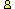CTK Exchange CTK Wiki Math Front Page Movie shortcuts Personal info Awards Terms of use Privacy Policy Cut The Knot! MSET99 Talk Games & Puzzles Arithmetic/Algebra Geometry Probability Eye Opener Analog Gadgets Inventor's Paradox Did you know?... Proofs Math as Language Things Impossible My Logo Math Poll Other Math sit's Guest book News sit's Recommend this siteCTK Exchange

 Subject: "H. Eves' Tricky Quickie In N-Gon" Previous Topic | Next Topic
 ConferencesThe CTK ExchangeCollege mathTopic #737Printer-friendly copyEmail this topic to a friend Reading Topic #737
Bui Quang Tuan
Member since Jun-23-07
Jul-29-10, 06:32 AM (EST)"H. Eves' Tricky Quickie In N-Gon"

 Dear Alex,Other than triangle, H. Eves' tricky quickie can be used for n gon. I recommend one better geometric inlustration for following page:https://www.cut-the-knot.org/Curriculum/Geometry/TouchingCircles.shtmlWe denote X(Y) as a circle centered at X passing Y.A1A2... An is any n-gonWe select on line AnA1 any point P1A circle A1(P1) cuts line A1A2 at P2A circle A2(P2) cuts line A2A3 at P3A circle A3(P3) cuts line A3A4 at P4...A circle An(Pn) cuts line AnA1 at Q1We finish construction of the first chain of circles with:n circles Ai(Pi), n points PiPoint Q1 is generally not P1 and use for starting point of second chain of circles:A circle A1(Q1) cuts line A1A2 at Q2A circle A2(Q2) cuts line A2A3 at Q3A circle A3(Q3) cuts line A3A4 at Q4...A circle An(Qn) cuts line AnA1 at RCase A: If n odd a). R=P1 and we can not make more another chain of circles.b). P1Q1 = P2Q2 = P3Q3 = ... = PnQn but changed when we change starting point P1.c). Midpoints Mi of segments PiQi are fixed with any starting point P1d). If we select P1 = M1 then Pi=Qi, Ai(Pi) = Ai(Qi) and they are sequence of circles as required in the problem. It is also geometric procedure to construct these circles.Case B: If n evena). R<>P1 and we can make chains of circles infinitely.b). P1Q1 = P2Q2 = P3Q3 = ... = PnQn = t = constant with any starting point P1c). Hence, we can see one pair of circles Ai(Pi)Ai(Qi) at vertex Ai as one circle with thickness t.d). If we change n-gon until thickness t=0 then we get n-gon which has sequence of circles as required in the problem. In this case, we can select any starting point P1.Please note that all results are already proved in explaining text of the applet. Here is only geometric inlustration by using H. Eves' tricky quickie.By the way: please check to see and correct page:https://www.cut-the-knot.org/geometry.shtmlSome items are empty, for example: item No. 860, item No. 780. I think it is because auto indexing.Best regards,Bui Quang Tuan

alexbCharter Member
2550 posts
Jul-29-10, 06:35 AM (EST)1. "RE: H. Eves' Tricky Quickie In N-Gon"
In response to message #0

 Thank you pointing out the typing mishaps and the good suggestion. I'll be looking into that.

 Conferences | Forums | Topics | Previous Topic | Next Topic
 Select another forum or conference Lobby The CTK Exchange (Conference)   |--Early math (Public)   |--Middle school (Public)   |--High school (Public)   |--College math (Public)   |--This and that (Public)   |--Guest book (Protected)   |--Thoughts and Suggestions (Public) Educational Press (Conference)   |--No Child Left Behind (Public)   |--Math Wars (Public)   |--Mathematics and general education (Public)You may be curious to have a look at the old CTK Exchange archive.